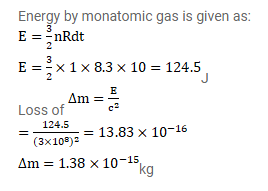# Find the loss in the mass of 1 mole of an ideal monatomic gas kept in a rigid

Question:

Find the loss in the mass of 1 mole of an ideal monatomic gas kept in a rigid container as it cools down by $10^{\circ} \mathrm{C}$. The gas constant $\mathrm{R}=8.3 \mathrm{~J} / \mathrm{mol}-\mathrm{K}$.

Solution: